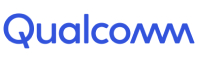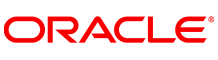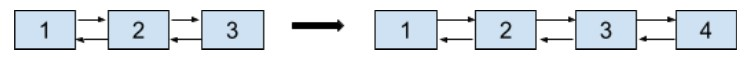New update is available. Click here to update.
Topics

# Insertion In Doubly Linked List

Easy0/40
Average time to solve is 15m+5 more companies

## Problem statement

You are given a Doubly linked list, where every node in the linked list contains two pointers ‘next’ and ‘prev’ which point to the next node and previous node in the list respectively. All nodes have some positive integer value associated with them. Your task is to insert an integer value ‘VAL’ in the linked list at a given position ‘K’.

Note:

``````The position given will always be less than or equal to the length of the linked list.
Assume that the Indexing for the linked list starts from 0.
``````
EXAMPLE:
``````Input :
‘K’ = 3, ‘VAL’ = 4
list = [1, 2, 3]
Output: [1, 2, 3, 4]
````````````The ‘VAL’ = 4, is inserted at end of the above doubly linked list.
``````
Detailed explanation ( Input/output format, Notes, Images )
Constraints :
``````1 <= 'T' <= 10
1 <= Length of the list <= 10^4
0 <= ‘K’ <= Length of the list, where ‘K’ is 0-indexed.
1 <= 'VAL' <= 10^5

Time Limit: 1 sec
``````
Sample Input 1:
``````2
0 0
1 2 3 4 -1
5 5
5 4 3 2 1 -1
``````
Sample Outpu.t 1:
``````0 1 2 3 4
5 4 3 2 1 5
``````
Explanation Of Sample Input 1 :
``````For the first test case, ‘K’ = 0 denotes the starting index of the list, so the new element is inserted before the head of the list and the new head will be then a node with ‘VAL’ = 0

Hence, the resulting doubly linked list would be : 0 1 2 3 4

For the second test case, ‘K’ = 5 = size of the list denotes the new element to be inserted at the end of the list.

Hence, the resulting doubly linked list would be: 5 4 3 2 1 5
``````
Sample Input 2 :
``````2
2 4
10 11 5 -1
1 5
2 8 -1
``````
Sample Output 2 :
``````10 11 4 5
2 5 8
``````Console